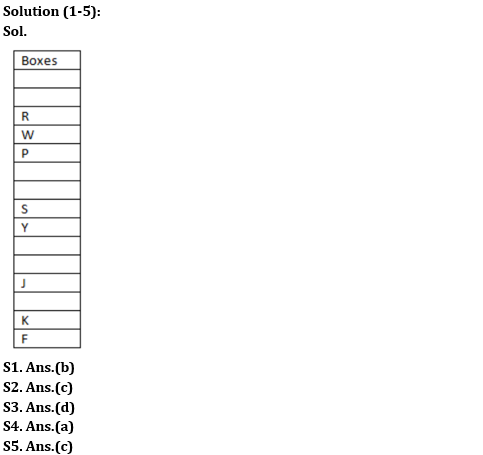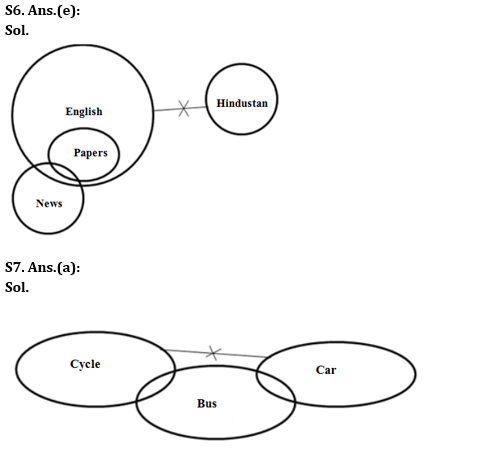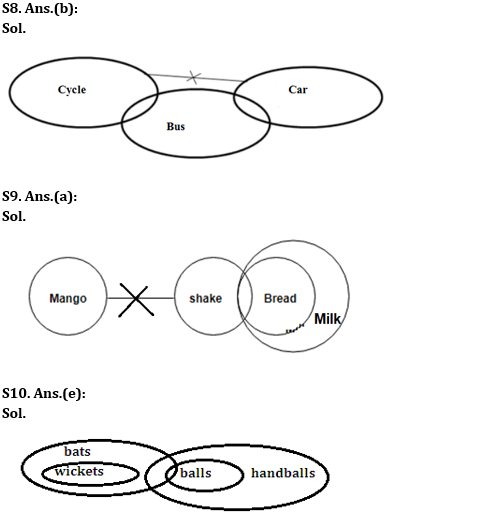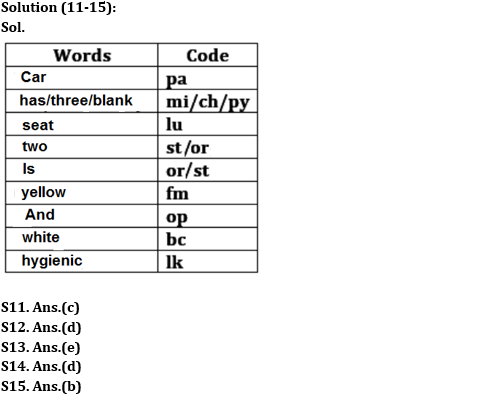Latest Banking jobs   »

# Reasoning Ability Quiz For RBI Grade B/ ECGC PO/ SIDBI Grade A Prelims 2022- 24th May

Directions (1-5): Study the following information carefully and answer the questions given below:
A certain number of boxes are kept one above the other. Three boxes are kept between box W and box S. Box S is kept exactly between box W and box J. Two boxes are kept between box J and box F which is kept below box J. Box K is kept just above box F. Two boxes are kept between box J and box Y. Box R is kept just above box W. Box R is third either from the topmost or from the bottommost position. One box is kept between box R and box P which is neither placed at the top nor at the bottom. Box K is kept at the second position from the bottom.

Q1. How many boxes are there in the given arrangement?
(a) Fourteen
(b) Fifteen
(c) Thirteen
(d) Sixteen
(e) None of these

Q2. Which among the following is kept at the bottommost position?
(a) Box W
(b) Box S
(c) Box F
(d) Box Y
(e) None

Q3. How many boxes are kept between box S and box R?
(a) One
(b) Two
(c) Five
(d) Four
(e) None of these

Q4. If box A is kept between box J and box K, then how many boxes are there between box A and box P?
(a) Seven
(b) Six
(c) Eight
(d) Nine
(e) Four

Q5. What is the position of box J in the given arrangement?
(a) Sixth from the bottom
(b) Fifth from the top
(c) Fourth from the bottom
(d) Seventh from the top
(e) None of these

Directions (6-10): In each question below, some statements are given followed by some conclusions. You have to take the given statements to be true even if they seem to be at variance with commonly known facts. Read all the conclusions and then decide which of the given conclusions logically follows/follow from the given statements, disregarding commonly known facts.

Q6. Statements: Only a few News are papers. No Hindustan is English.All Papers are English.
Conclusions:
I. All English being News is a possibility.
II. All English being Papers is a possibility.
(a) If only conclusion I follows.
(b) If only conclusion II follows.
(c) If either conclusion I or II follows.
(d) If neither conclusion I nor II follows.
(e) If both conclusions I and II follow

Q7. Statements: Only a few Cycles are Buses. No car is a cycle. Only a few cars are buses.
Conclusions:
I. Some cycles are definitely not buses.
II. All buses being cars is a possibility.
(a) If only conclusion I follows.
(b) If only conclusion II follows.
(c) If either conclusion I or II follows.
(d) If neither conclusion I nor II follows.
(e) If both conclusions I and II follow.

Q8. Statements: Only a few Cycles are Buses. No car is a cycle. Only a few cars are buses..
Conclusions:
I. All bus being cycle is a possibility.
II. Some cars are definitely not buses.
(a) If only conclusion I follows.
(b) If only conclusion II follows.
(c) If either conclusion I or II follows.
(d) If neither conclusion I nor II follows.
(e) If both conclusions I and II follow.

Q9. Statements: All Breads are Milk. Some Breads are Shake. No Shake is a Mango.
Conclusions:
II. At least some Mangoes are not milk.
(a) If only conclusion I follows.
(b) If only conclusion II follows.
(c) If either conclusion I or II follows.
(d) If neither conclusion I nor II follows.
(e) If both conclusions I and II follow.

Q10. Statements: All balls are handballs. Some bats are balls. All wickets are bats.
Conclusions:
I. All handballs being bats is a possibility.
II. All wickets can be balls
(a) If only conclusion I follows.
(b) If only conclusion II follows.
(c) If either conclusion I or II follows.
(d) If neither conclusion I nor II follows.
(e) If both conclusions I and II follow.

Directions (11-15): Study the information given below and answer the questions based on it.
In the certain code language,
“Car has three seat blank” is written as “lu ch mi pa py”.
“Two is yellow seat” is written as “fm or lu st”.
“Yellow and white car” is written as “op bc pa fm”.
“White two is hygienic” is written as “st lk or bc”.

Q11. Which of the following is the code for “seat”?
(a) or
(b) bc
(c) lu
(d) op
(e) lk

Q12. What does “op” stand for?
(a) Yellow
(b) White
(c) Car
(d) And
(e) Is

Q13. What is the code for “seat is hygienic”?
(a) lu or lk
(b) lu st lk
(c) st or bc
(d) bc lk lu
(e) Either (a) or (b)

Q14. How is “three” written in the code language?
(a) mi
(b) pa
(c) bc
(d) Can’t be determined
(e) lu

Q15. “or fm lu st” is the code for which of the following?
(a) and car seat has
(b) Two seat is yellow
(c) Two is three seat
(d) Can’t be determined
(e) None of these

Solutions#### Congratulations!